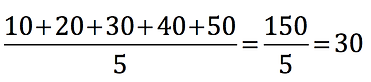top of page

## Chapter 5 - Day 2 - Lesson 5.2

##### Learning Targets
• Make a histogram to display the probability distribution of a discrete random variable and describe its shape.

• Calculate and interpret the mean (expected value) of a discrete random variable.

• Calculate and interpret the standard deviation of a discrete random variable.

##### Activity: Who will win in skee ball?

In this activity, students must predict which Stats Medic will win the Skee Ball game, based on probability and expected value.  We really like this activity because students will get contradictory evidence as they work through the activity, ultimately revealing a need for expected value.  Question #3 suggests that Mr. Wilcox will win.  Question #4 likely shows that they will tie.  Question #5 should reveal that Ms. Gallas is more likely to win (true story).

Question #4 is written with the intention that students will do the wrong calculation for the mean.  Almost all of our students reverted to the one formula for mean with which they are familiar (add up all the values and divide by 5).

##### Why do we need weighted means?

In Chapter 1 when we calculated a mean, we simply added up all the values and divided by the total number of values.  In this context, we would get a mean of:This works great if each of the values is equally likely to occur.  But we know from the probability table that each of these values are not equally likely.  Some of the values occur more frequently than others and should be weighted more.  We do this by calculating a weighted mean:It is important to note that back in Chapter 1 when we were calculating means, we were in fact using weighted means.  It just so happens that because all of the values are equally likely to occur, they all have a probability of 1/5 = 0.20.  So when we divided the total by 5, we were really just weighting each value by 0.20:##### Teaching Tip:

Students can use the Applet or a TI calculator to get the standard deviation of a discrete random variable, rather than calculating it by hand using the formula.  Regardless of which method your students are using to get the standard deviation, it is more important that they can interpret the value.  In our Skee Ball context, a randomly selected score from Mr. Wilcox “typically differs from the mean” of 22.6 by 12.777 points.

bottom of page